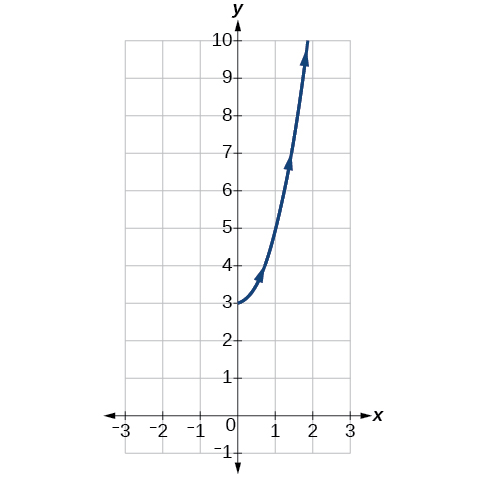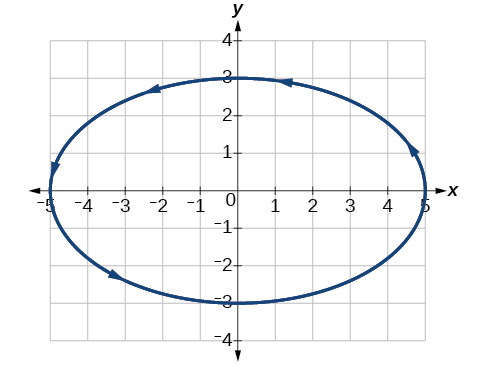# 8.7 Parametric equations: graphs

 Page 1 / 4
In this section you will:
• Graph plane curves described by parametric equations by plotting points.
• Graph parametric equations.

It is the bottom of the ninth inning, with two outs and two men on base. The home team is losing by two runs. The batter swings and hits the baseball at 140 feet per second and at an angle of approximately $\text{\hspace{0.17em}}45°\text{\hspace{0.17em}}$ to the horizontal. How far will the ball travel? Will it clear the fence for a game-winning home run? The outcome may depend partly on other factors (for example, the wind), but mathematicians can model the path of a projectile and predict approximately how far it will travel using parametric equations . In this section, we’ll discuss parametric equations and some common applications, such as projectile motion problems.Parametric equations can model the path of a projectile. (credit: Paul Kreher, Flickr)

## Graphing parametric equations by plotting points

In lieu of a graphing calculator or a computer graphing program, plotting points to represent the graph of an equation is the standard method. As long as we are careful in calculating the values, point-plotting is highly dependable.

Given a pair of parametric equations, sketch a graph by plotting points.

1. Construct a table with three columns: $\text{\hspace{0.17em}}t,x\left(t\right),\text{and}\text{\hspace{0.17em}}\text{\hspace{0.17em}}y\left(t\right).$
2. Evaluate $x$ and $y$ for values of $t$ over the interval for which the functions are defined.
3. Plot the resulting pairs $\text{\hspace{0.17em}}\left(x,y\right).$

## Sketching the graph of a pair of parametric equations by plotting points

Sketch the graph of the parametric equations $x\left(t\right)={t}^{2}+1,\text{\hspace{0.17em}}\text{\hspace{0.17em}}y\left(t\right)=2+t.$

Construct a table of values for $\text{\hspace{0.17em}}t,x\left(t\right),\text{\hspace{0.17em}}$ and $\text{\hspace{0.17em}}y\left(t\right),\text{\hspace{0.17em}}$ as in [link] , and plot the points in a plane.

$t$ $x\left(t\right)={t}^{2}+1$ $y\left(t\right)=2+t$
$-5$ $26$ $-3$
$-4$ $17$ $-2$
$-3$ $10$ $-1$
$-2$ $5$ $0$
$-1$ $2$ $1$
$0$ $1$ $2$
$1$ $2$ $3$
$2$ $5$ $4$
$3$ $10$ $5$
$4$ $17$ $6$
$5$ $26$ $7$

The graph is a parabola with vertex at the point $\text{\hspace{0.17em}}\left(1,2\right),$ opening to the right. See [link] .

Sketch the graph of the parametric equations $\text{\hspace{0.17em}}x=\sqrt{t},\text{\hspace{0.17em}}\text{\hspace{0.17em}}y=2t+3,\text{\hspace{0.17em}}\text{\hspace{0.17em}}\text{\hspace{0.17em}}0\le t\le 3.$## Sketching the graph of trigonometric parametric equations

Construct a table of values for the given parametric equations and sketch the graph:

$\begin{array}{l}\\ \begin{array}{l}x=2\mathrm{cos}\text{\hspace{0.17em}}t\hfill \\ y=4\mathrm{sin}\text{\hspace{0.17em}}t\hfill \end{array}\end{array}$

Construct a table like that in [link] using angle measure in radians as inputs for $\text{\hspace{0.17em}}t,\text{\hspace{0.17em}}$ and evaluating $\text{\hspace{0.17em}}x\text{\hspace{0.17em}}$ and $\text{\hspace{0.17em}}y.\text{\hspace{0.17em}}$ Using angles with known sine and cosine values for $\text{\hspace{0.17em}}t\text{\hspace{0.17em}}$ makes calculations easier.

$t$ $x=2\mathrm{cos}\text{\hspace{0.17em}}t$ $y=4\mathrm{sin}\text{\hspace{0.17em}}t$
0 $x=2\mathrm{cos}\left(0\right)=2$ $y=4\mathrm{sin}\left(0\right)=0$
$\frac{\pi }{6}$ $x=2\mathrm{cos}\left(\frac{\pi }{6}\right)=\sqrt{3}$ $y=4\mathrm{sin}\left(\frac{\pi }{6}\right)=2$
$\frac{\pi }{3}$ $x=2\mathrm{cos}\left(\frac{\pi }{3}\right)=1$ $y=4\mathrm{sin}\left(\frac{\pi }{3}\right)=2\sqrt{3}$
$\frac{\pi }{2}$ $x=2\mathrm{cos}\left(\frac{\pi }{2}\right)=0$ $y=4\mathrm{sin}\left(\frac{\pi }{2}\right)=4$
$\frac{2\pi }{3}$ $x=2\mathrm{cos}\left(\frac{2\pi }{3}\right)=-1$ $y=4\mathrm{sin}\left(\frac{2\pi }{3}\right)=2\sqrt{3}$
$\frac{5\pi }{6}$ $x=2\mathrm{cos}\left(\frac{5\pi }{6}\right)=-\sqrt{3}$ $y=4\mathrm{sin}\left(\frac{5\pi }{6}\right)=2$
$\pi$ $x=2\mathrm{cos}\left(\pi \right)=-2$ $y=4\mathrm{sin}\left(\pi \right)=0$
$\frac{7\pi }{6}$ $x=2\mathrm{cos}\left(\frac{7\pi }{6}\right)=-\sqrt{3}$ $y=4\mathrm{sin}\left(\frac{7\pi }{6}\right)=-2$
$\frac{4\pi }{3}$ $x=2\mathrm{cos}\left(\frac{4\pi }{3}\right)=-1$ $y=4\mathrm{sin}\left(\frac{4\pi }{3}\right)=-2\sqrt{3}$
$\frac{3\pi }{2}$ $x=2\mathrm{cos}\left(\frac{3\pi }{2}\right)=0$ $y=4\mathrm{sin}\left(\frac{3\pi }{2}\right)=-4$
$\frac{5\pi }{3}$ $x=2\mathrm{cos}\left(\frac{5\pi }{3}\right)=1$ $y=4\mathrm{sin}\left(\frac{5\pi }{3}\right)=-2\sqrt{3}$
$\frac{11\pi }{6}$ $x=2\mathrm{cos}\left(\frac{11\pi }{6}\right)=\sqrt{3}$ $y=4\mathrm{sin}\left(\frac{11\pi }{6}\right)=-2$
$2\pi$ $x=2\mathrm{cos}\left(2\pi \right)=2$ $y=4\mathrm{sin}\left(2\pi \right)=0$

By the symmetry shown in the values of $x$ and $\text{\hspace{0.17em}}y,\text{\hspace{0.17em}}$ we see that the parametric equations represent an ellipse . The ellipse is mapped in a counterclockwise direction as shown by the arrows indicating increasing $\text{\hspace{0.17em}}t\text{\hspace{0.17em}}$ values.

Graph the parametric equations: $\text{\hspace{0.17em}}x=5\mathrm{cos}\text{\hspace{0.17em}}t,\text{\hspace{0.17em}}\text{\hspace{0.17em}}y=3\mathrm{sin}\text{\hspace{0.17em}}t.$## Graphing parametric equations and rectangular form together

Graph the parametric equations $\text{\hspace{0.17em}}x=5\mathrm{cos}\text{\hspace{0.17em}}t\text{\hspace{0.17em}}$ and $\text{\hspace{0.17em}}y=2\mathrm{sin}\text{\hspace{0.17em}}t.\text{\hspace{0.17em}}$ First, construct the graph using data points generated from the parametric form . Then graph the rectangular form of the equation. Compare the two graphs.

Construct a table of values like that in [link] .

$t$ $x=5\mathrm{cos}\text{\hspace{0.17em}}t$ $y=2\mathrm{sin}\text{\hspace{0.17em}}t$
$\text{0}$ $x=5\mathrm{cos}\left(0\right)=5$ $y=2\mathrm{sin}\left(0\right)=0$
$\text{1}$ $x=5\mathrm{cos}\left(1\right)\approx 2.7$ $y=2\mathrm{sin}\left(1\right)\approx 1.7$
$\text{2}$ $x=5\mathrm{cos}\left(2\right)\approx -2.1$ $y=2\mathrm{sin}\left(2\right)\approx 1.8$
$\text{3}$ $x=5\mathrm{cos}\left(3\right)\approx -4.95$ $y=2\mathrm{sin}\left(3\right)\approx 0.28$
$\text{4}$ $x=5\mathrm{cos}\left(4\right)\approx -3.3$ $y=2\mathrm{sin}\left(4\right)\approx -1.5$
$\text{5}$ $x=5\mathrm{cos}\left(5\right)\approx 1.4$ $y=2\mathrm{sin}\left(5\right)\approx -1.9$
$-1$ $x=5\mathrm{cos}\left(-1\right)\approx 2.7$ $y=2\mathrm{sin}\left(-1\right)\approx -1.7$
$-2$ $x=5\mathrm{cos}\left(-2\right)\approx -2.1$ $y=2\mathrm{sin}\left(-2\right)\approx -1.8$
$-3$ $x=5\mathrm{cos}\left(-3\right)\approx -4.95$ $y=2\mathrm{sin}\left(-3\right)\approx -0.28$
$-4$ $x=5\mathrm{cos}\left(-4\right)\approx -3.3$ $y=2\mathrm{sin}\left(-4\right)\approx 1.5$
$-5$ $x=5\mathrm{cos}\left(-5\right)\approx 1.4$ $y=2\mathrm{sin}\left(-5\right)\approx 1.9$

Plot the $\text{\hspace{0.17em}}\left(x,y\right)\text{\hspace{0.17em}}$ values from the table. See [link] .

Next, translate the parametric equations to rectangular form. To do this, we solve for $\text{\hspace{0.17em}}t\text{\hspace{0.17em}}$ in either $\text{\hspace{0.17em}}x\left(t\right)\text{\hspace{0.17em}}$ or $\text{\hspace{0.17em}}y\left(t\right),\text{\hspace{0.17em}}$ and then substitute the expression for $\text{\hspace{0.17em}}t\text{\hspace{0.17em}}$ in the other equation. The result will be a function $y\left(x\right)$ if solving for $\text{\hspace{0.17em}}t\text{\hspace{0.17em}}$ as a function of $\text{\hspace{0.17em}}x,\text{\hspace{0.17em}}$ or $\text{\hspace{0.17em}}x\left(y\right)$ if solving for $\text{\hspace{0.17em}}t\text{\hspace{0.17em}}$ as a function of $\text{\hspace{0.17em}}y.\text{\hspace{0.17em}}$

Then, use the Pythagorean Theorem .

$\begin{array}{r}\hfill {\mathrm{cos}}^{2}t+{\mathrm{sin}}^{2}t=1\\ \hfill {\left(\frac{x}{5}\right)}^{2}+{\left(\frac{y}{2}\right)}^{2}=1\\ \hfill \frac{{x}^{2}}{25}+\frac{{y}^{2}}{4}=1\end{array}$

can you not take the square root of a negative number
No because a negative times a negative is a positive. No matter what you do you can never multiply the same number by itself and end with a negative
lurverkitten
Actually you can. you get what's called an Imaginary number denoted by i which is represented on the complex plane. The reply above would be correct if we were still confined to the "real" number line.
Liam
Suppose P= {-3,1,3} Q={-3,-2-1} and R= {-2,2,3}.what is the intersection
can I get some pretty basic questions
In what way does set notation relate to function notation
Ama
is precalculus needed to take caculus
It depends on what you already know. Just test yourself with some precalculus questions. If you find them easy, you're good to go.
Spiro
the solution doesn't seem right for this problem
what is the domain of f(x)=x-4/x^2-2x-15 then
x is different from -5&3
Seid
All real x except 5 and - 3
Spiro
***youtu.be/ESxOXfh2Poc
Loree
how to prroved cos⁴x-sin⁴x= cos²x-sin²x are equal
Don't think that you can.
Elliott
By using some imaginary no.
Tanmay
how do you provided cos⁴x-sin⁴x = cos²x-sin²x are equal
What are the question marks for?
Elliott
Someone should please solve it for me Add 2over ×+3 +y-4 over 5 simplify (×+a)with square root of two -×root 2 all over a multiply 1over ×-y{(×-y)(×+y)} over ×y
For the first question, I got (3y-2)/15 Second one, I got Root 2 Third one, I got 1/(y to the fourth power) I dont if it's right cause I can barely understand the question.
Is under distribute property, inverse function, algebra and addition and multiplication function; so is a combined question
Abena
find the equation of the line if m=3, and b=-2
graph the following linear equation using intercepts method. 2x+y=4
Ashley
how
Wargod
what?
John
ok, one moment
UriEl
how do I post your graph for you?
UriEl
it won't let me send an image?
UriEl
also for the first one... y=mx+b so.... y=3x-2
UriEl
y=mx+b you were already given the 'm' and 'b'. so.. y=3x-2
Tommy
Please were did you get y=mx+b from
Abena
y=mx+b is the formula of a straight line. where m = the slope & b = where the line crosses the y-axis. In this case, being that the "m" and "b", are given, all you have to do is plug them into the formula to complete the equation.
Tommy
thanks Tommy
Nimo
0=3x-2 2=3x x=3/2 then . y=3/2X-2 I think
Given
co ordinates for x x=0,(-2,0) x=1,(1,1) x=2,(2,4)
neil
"7"has an open circle and "10"has a filled in circle who can I have a set builder notation
Where do the rays point?
Spiro
x=-b+_Гb2-(4ac) ______________ 2a
I've run into this: x = r*cos(angle1 + angle2) Which expands to: x = r(cos(angle1)*cos(angle2) - sin(angle1)*sin(angle2)) The r value confuses me here, because distributing it makes: (r*cos(angle2))(cos(angle1) - (r*sin(angle2))(sin(angle1)) How does this make sense? Why does the r distribute once
so good
abdikarin
this is an identity when 2 adding two angles within a cosine. it's called the cosine sum formula. there is also a different formula when cosine has an angle minus another angle it's called the sum and difference formulas and they are under any list of trig identities
strategies to form the general term
carlmark
consider r(a+b) = ra + rb. The a and b are the trig identity.
Mike
How can you tell what type of parent function a graph is ?
generally by how the graph looks and understanding what the base parent functions look like and perform on a graph
William
if you have a graphed line, you can have an idea by how the directions of the line turns, i.e. negative, positive, zero
William
y=x will obviously be a straight line with a zero slope
William
y=x^2 will have a parabolic line opening to positive infinity on both sides of the y axis vice versa with y=-x^2 you'll have both ends of the parabolic line pointing downward heading to negative infinity on both sides of the y axis
William
y=x will be a straight line, but it will have a slope of one. Remember, if y=1 then x=1, so for every unit you rise you move over positively one unit. To get a straight line with a slope of 0, set y=1 or any integer.
Aaron
yes, correction on my end, I meant slope of 1 instead of slope of 0
William
what is f(x)=
I don't understand
Joe
Typically a function 'f' will take 'x' as input, and produce 'y' as output. As 'f(x)=y'. According to Google, "The range of a function is the complete set of all possible resulting values of the dependent variable (y, usually), after we have substituted the domain."
Thomas
Sorry, I don't know where the "Â"s came from. They shouldn't be there. Just ignore them. :-)
Thomas
Darius
Thanks.
Thomas
Â
Thomas
It is the Â that should not be there. It doesn't seem to show if encloses in quotation marks. "Â" or 'Â' ... Â
Thomas
Now it shows, go figure?
ThomasByBy OpenStaxBy Michael SagBy Donyea SweetsBy Vongkol HENGBy Brooke DelaneyBy OpenStaxByBy Jonathan LongBy P. Wynn NormanBy OpenStax